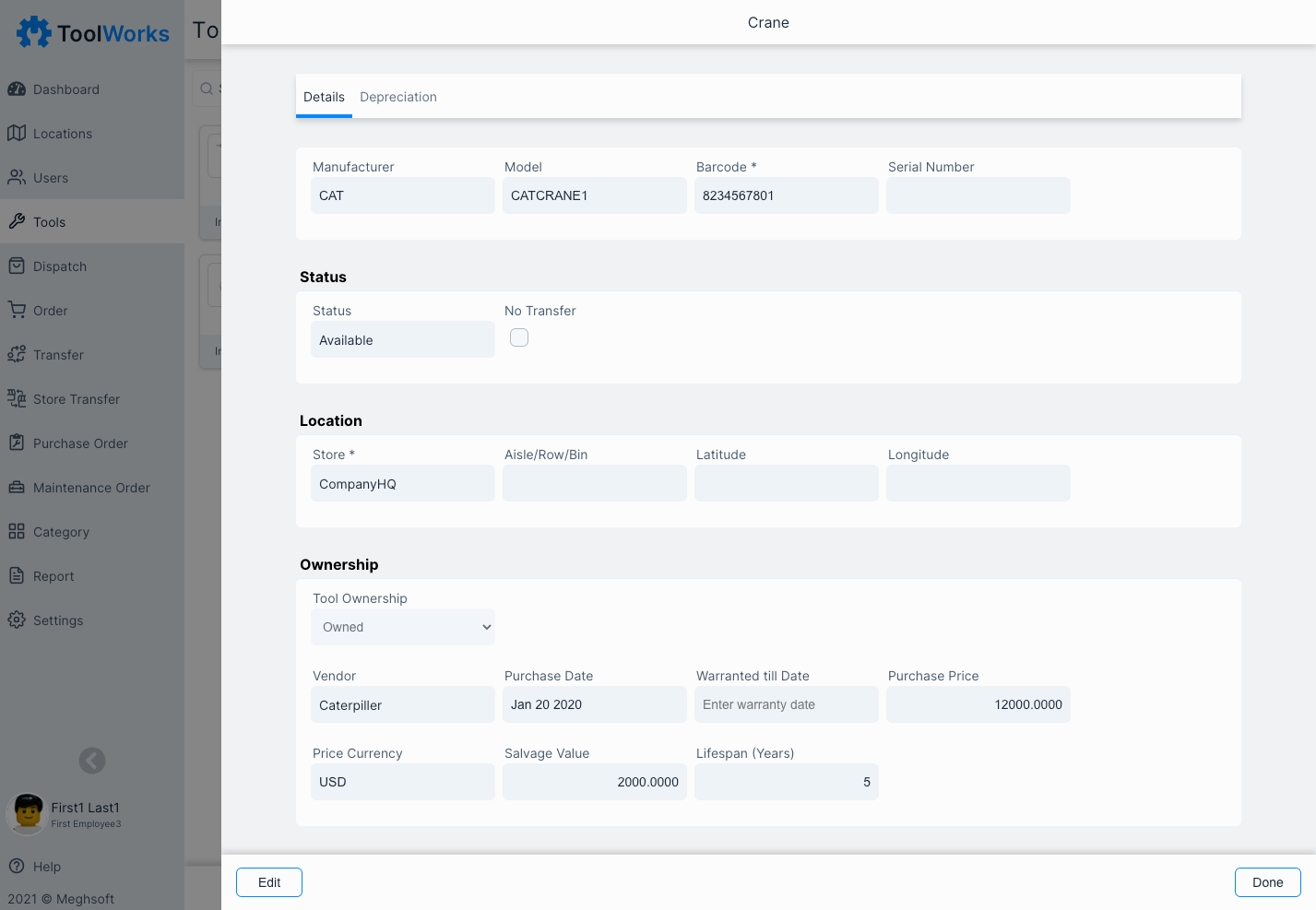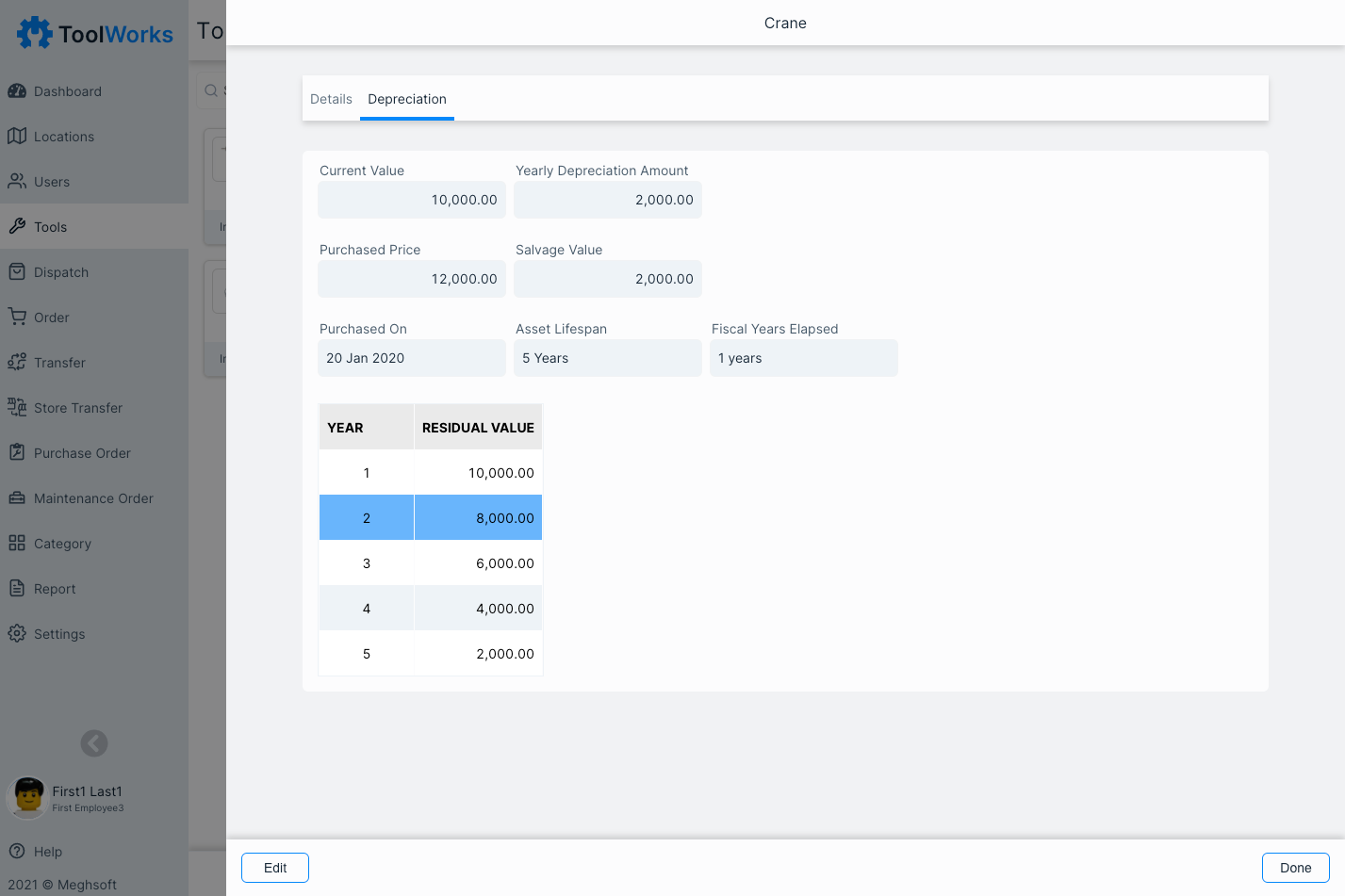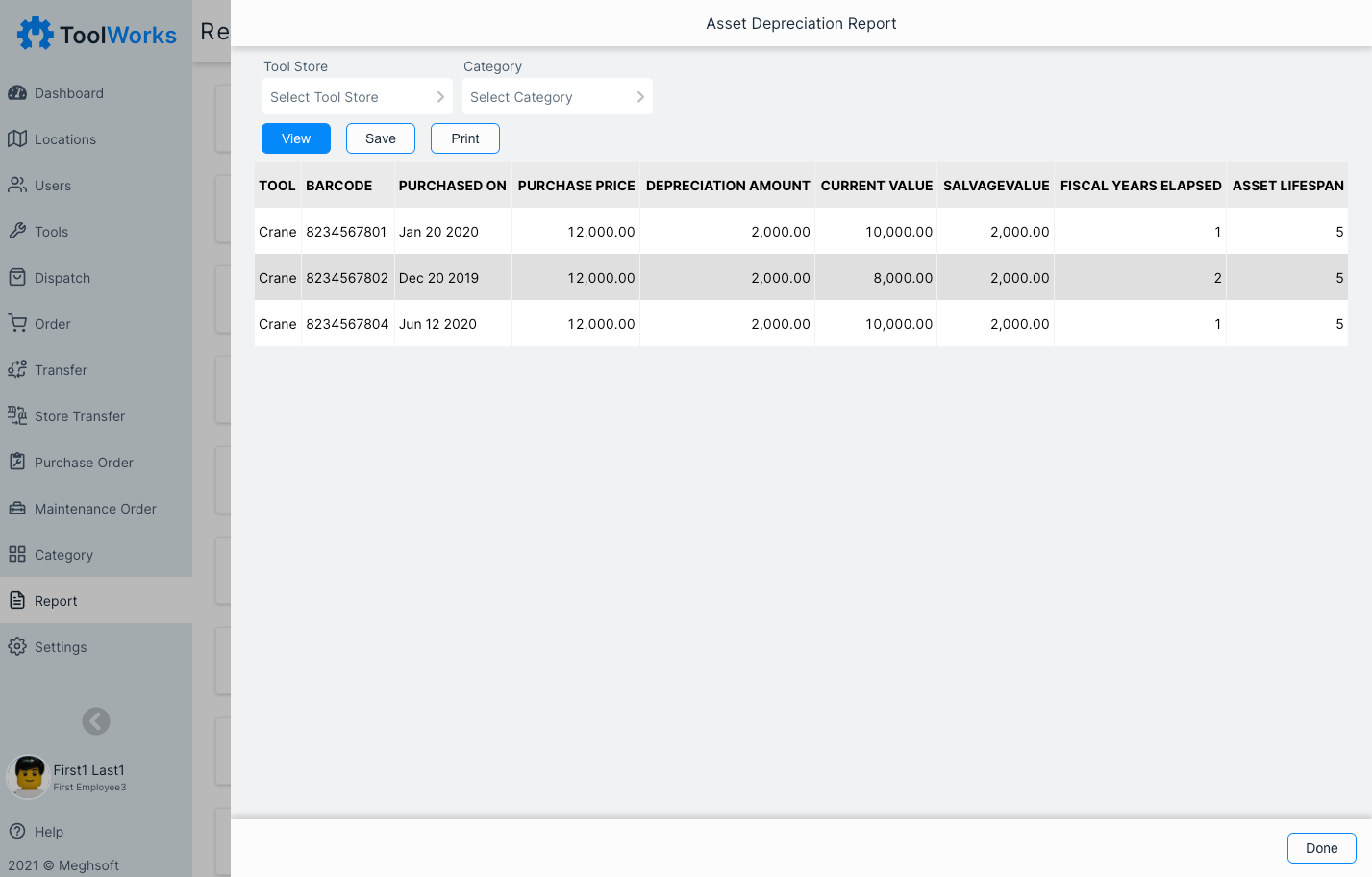# Asset Depreciation

Whatever the size of your business, long-term assets like tools and equipment can be big investment and need to be accounted for both accounting and tax purposes.

ToolWorks includes automatic asset depreciation calculations for Tools and Equipments, as well as support for Fiscal Year.

Get Started

## What is asset depreciation?

Depreciation is a key component in understanding your business's profitability and is recorded on the income and balance statements. Depreciation is the decrease in the value of assets and the method used to reallocate, or "write down" the cost of a tangible asset over its useful life span.

## Straight-line Depreciation

Straight line depreciation is a common method of depreciation where the value of a fixed asset is reduced gradually over its useful life. Each full accounting year will be allocated the same amount of the percentage of asset’s cost when you are using the straight-line method of depreciation.In order to calculate depreciation using this method, you will need the following three inputs:

1. Useful Lifespan of asset

The useful life is the time period over which the organization or company considers the fixed asset to be productive. The useful life is measured in years.

2. Cost of Asset

The asset cost is the total price of the asset

3. Salvage Value of asset

When a fixed asset comes to the end of its useful life and is fully depreciated, the company may continue to use it or consider selling it at a reduced amount. This is known as the salvage value of the asset.

Formula

Straight Line Annual Deprecation = ( Cost of Asset - Salvage Value of asset ) / Useful Lifespan of asset

## Example

Lets say you purchased a new equipment. Equipment Costs \$12,000 and it is expected to have salvage value of \$2,000 and useful life of 5 years.

Given these assumptions, its annual depreciation expense is: ( 12000 - 2000 ) / 5 = 2000## Report

Asset Depreciation report show depreciation for list of assets.The straight-line depreciation method allows you to use your tool and equipment and spread the cost across the time you decide to use it. Once you have calculated the depreciation expense of your assets, you’ll know how much of the asset’s total cost should be expensed each financial period.

### Explore Other Features

View All Features >